OpenCMISS-Iron Internal API Documentation
Regions

Regions are one of the primary objects in openCMISS. Regions are hierarchical in nature in that a region can have one parent region and a number of daughter sub-regions. Daughter regions are related in space to parent regions by an origin and an orientation of the regions coordinate system. Daughter regions may only have the same or fewer dimensions as the parent region. There is a global (world) region (number 0) that has the global (world) coordinate system.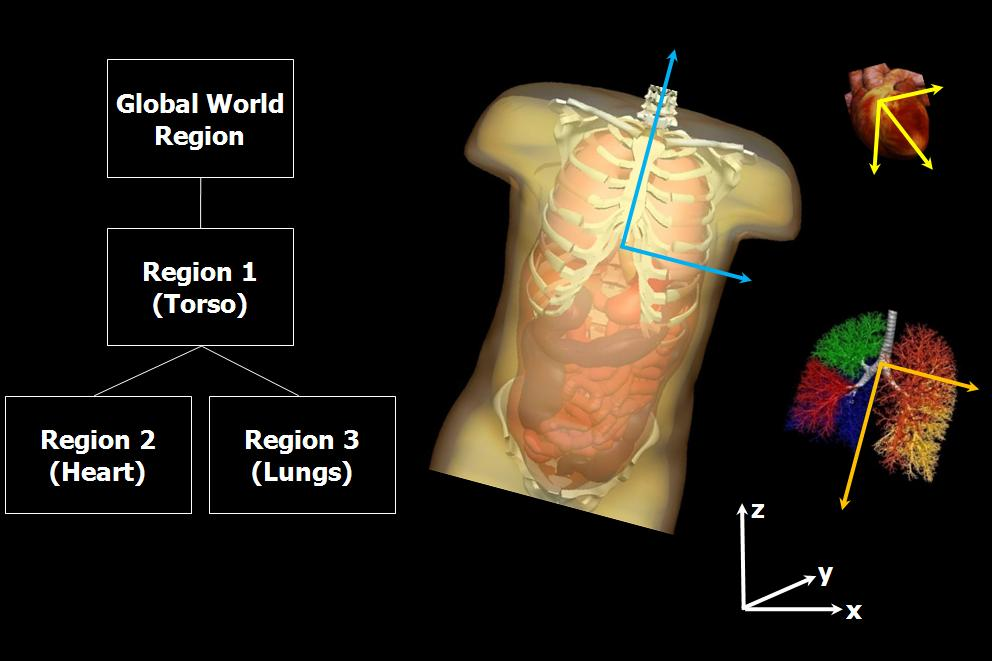Region has the following attributes

• User number
• Finished tag
• Label
• Number of sub(daughter) regions
• Coordinate system pointer
• Nodes
• Meshes
• Fields
• Equations
• Parent region pointer
• Daughter regions pointers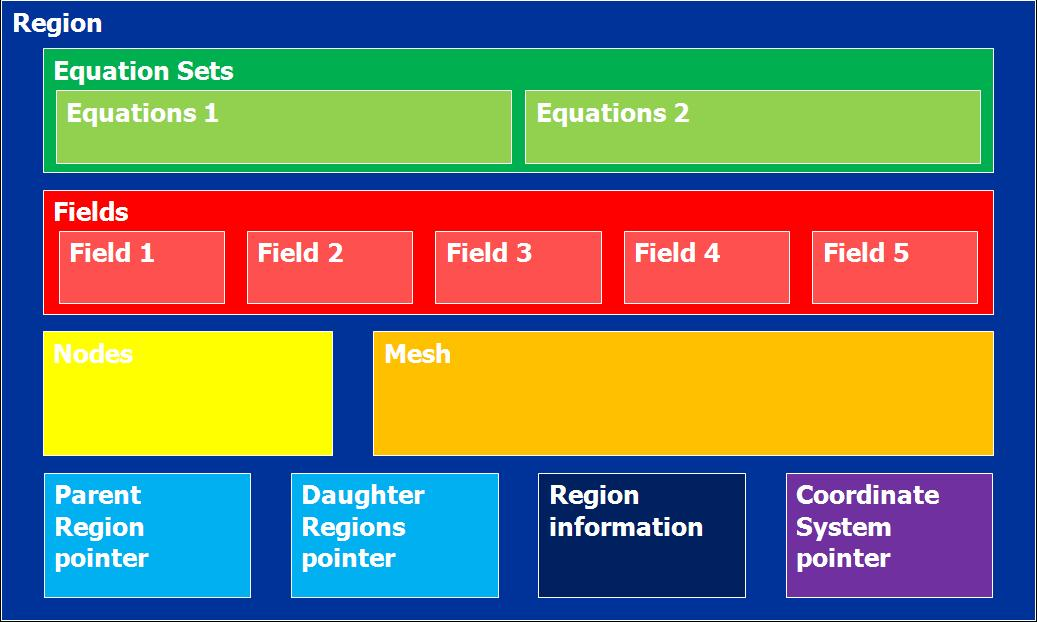# Nodes

There are three places storing nodal information. Nodes associated with region defines the nodes identification and the nodes geometric (initial) position.

Node has the following attributes

• User number
• Global number
• Label
• Initial Position

# Meshes

Meshes are topological constructs within a region which fields use to define themselves. Meshes are made up of a number of mesh components. All mesh components in a mesh must "conform", that is have the same number of elements, Xi directions etc.

Mesh has the following attributes:

• User number
• Global number
• Finished tag
• Region pointer
• Number of dimensions
• Number of components
• Embedded flag
• Embedding mesh pointer
• Embedded meshes pointers
• Number of elements
• Number of faces
• Number of lines
• Mesh topology pointers
• Decomposition pointers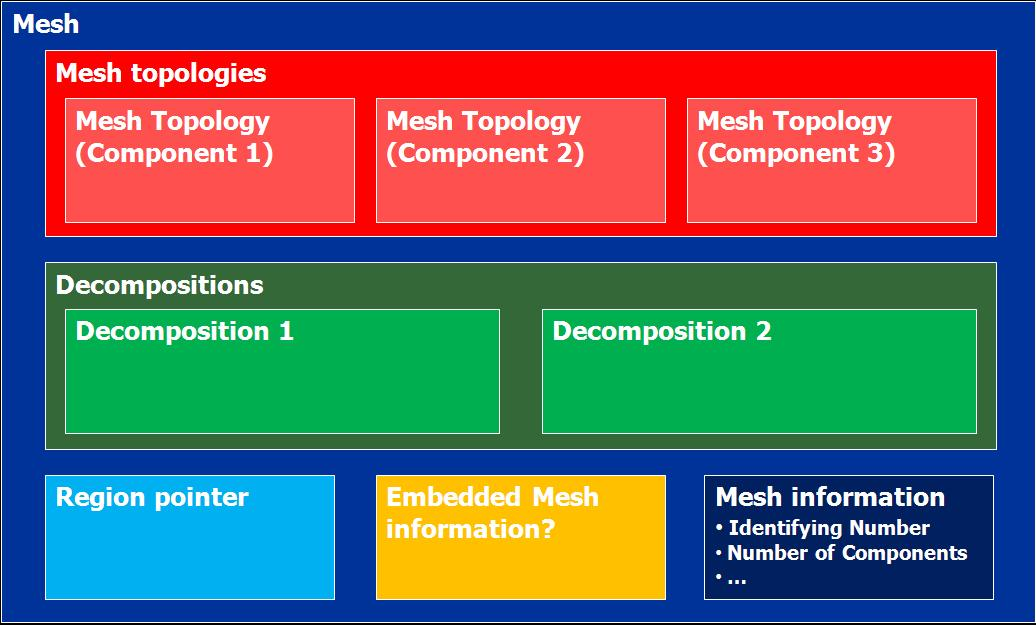## Mesh Topology

Mesh components (Topology) are made up from nodes, elements and basis functions. A new mesh component is required for each different form of interpolation e.g., one mesh component is bilinear Lagrange and another is biquadratic Lagrange.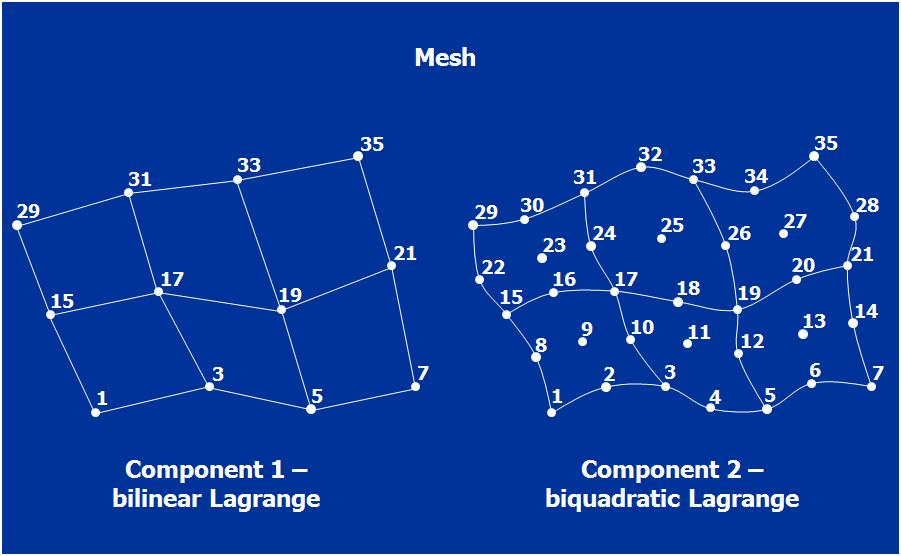Mesh topology has the following attributes:

• Mesh component number
• Mesh pointer
• Nodes pointers
• Element pointers
• DOFs pointers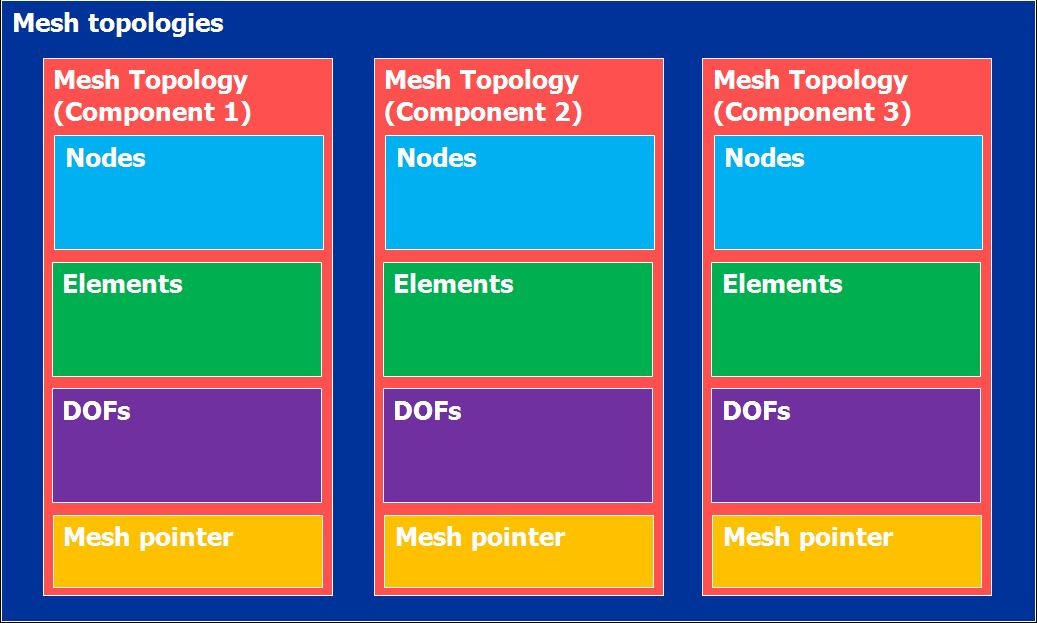## Decompositions

Mesh decomposition (partitioning) is used to split a computationally expensive mesh into smaller subdomains (parts) for parallel computing.

Decomposition has the following attributes

• User number
• Global number
• Finished tag
• Mesh pointer
• Mesh component number
• Decomposition type
• Number of domains
• Number of edge cut
• Element domain numbers
• Decomposition topology pointer
• Domains pointers(list of domain which has the same size as the number of components in the mesh)### Domain

Each domain stores domain information for relevant mesh component.

The domain object contains the following attributes:

• Decomposition pointer
• Mesh pointer
• Mesh component number
• Region pointer
• Number of dimensions
• Node domain(The domain number that the np'th global node is in for the domain decomposition. Note: the domain numbers start at 0 and go up to the NUMBER_OF_DOMAINS-1)
• Domain mappings(for each mapped object e.g. nodes, elements, etc)
• Domain topology pointer(elements, nodes, DOFs)#### Domain Mappings

Stores information for each mapped object e.g. nodes, elements, etc.

The domain mapping contains the following attributes:

• Number of local
• Total number of local
• Numbers of domain local
• Number of global
• Number of domains
• Number of internal
• Internal list
• Number of boundary
• Boundary list
• Number of ghost
• Ghost list
• Local to global map
• Global to local map
• Number of adjacent domains
• Pointer to list of adjacent domains by domain number
• List of adjacent domains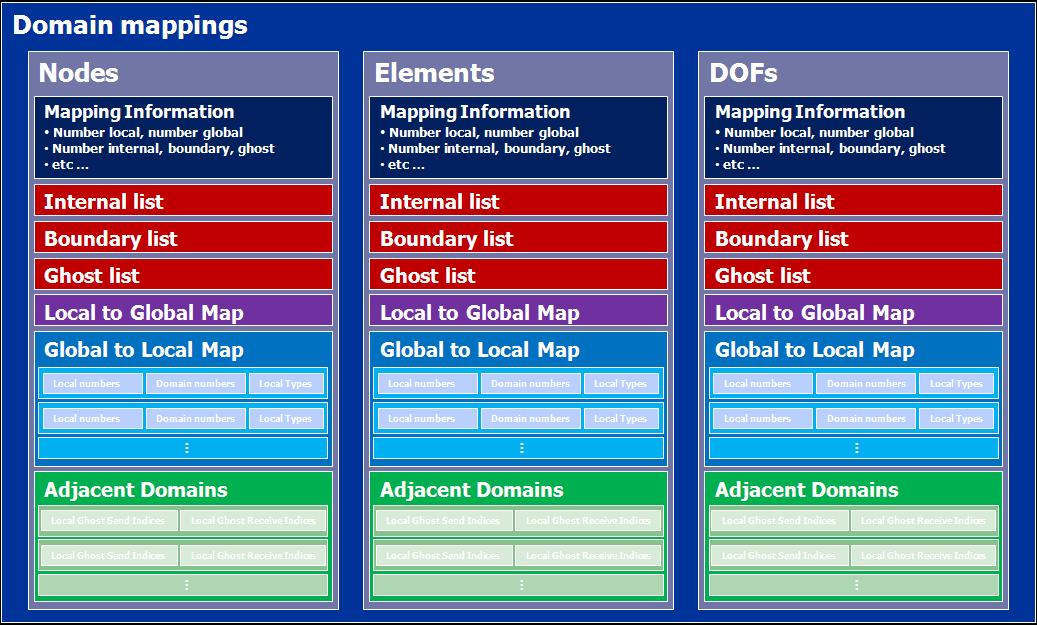# Fields

Fields are the central object for storing information and framing the problem. Fields have a number of field variables i.e., u, du/dn, du/dt, d2u/dt2. Each field variable has a number of components. A field is defined on a decomposed mesh. Each field variable component is defined on a decomposed mesh component.

Field can contains the following attributes:

• User number
• Global number
• Finished tag
• Region pointer
• Type(Geometric, Fibre, General, Material, Source)
• Dependent type(Independent, Dependent)
• Dimension
• Decomposition pointer
• Number of variables
• Variables
• Scalings sets
• Mappings(DOF->Field parameters)
• Parameter sets(distributed vectors)
• Geometric field pointer
• Geomatric field parameters
• Create values cache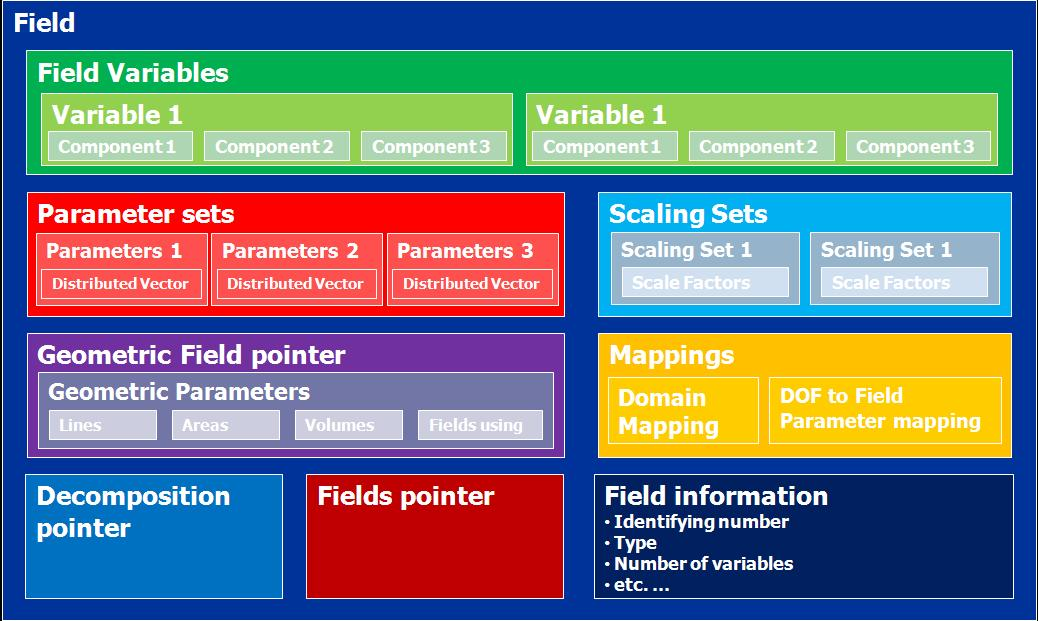## Field variable

Field variable stores variables for the field such as dependent variables. For example, in the Laplace's equation(FEM), it stores two variables: u and du/dn. Each field variable has a number of components.

Field variable has the following attributes:

• Variable number
• Variable type
• Field pointer
• Region pointer
• Max number of interpolation parameters
• Number of DOFs
• Total number of DOFs
• Global DOF List
• Domain mapping pointer
• Number of components
• Components

### Field Variable Component

Field Variable Component has the following attributes:

• Component number
• Variable pointer
• Field pointer
• Interpolation type
• Mesh component number
• Scaling index
• Domain pointer
• Max number of interpolation parameters
• Mappings(Field paramters->DOF)

## Parameter set

Parameter set stores values for each field variable component.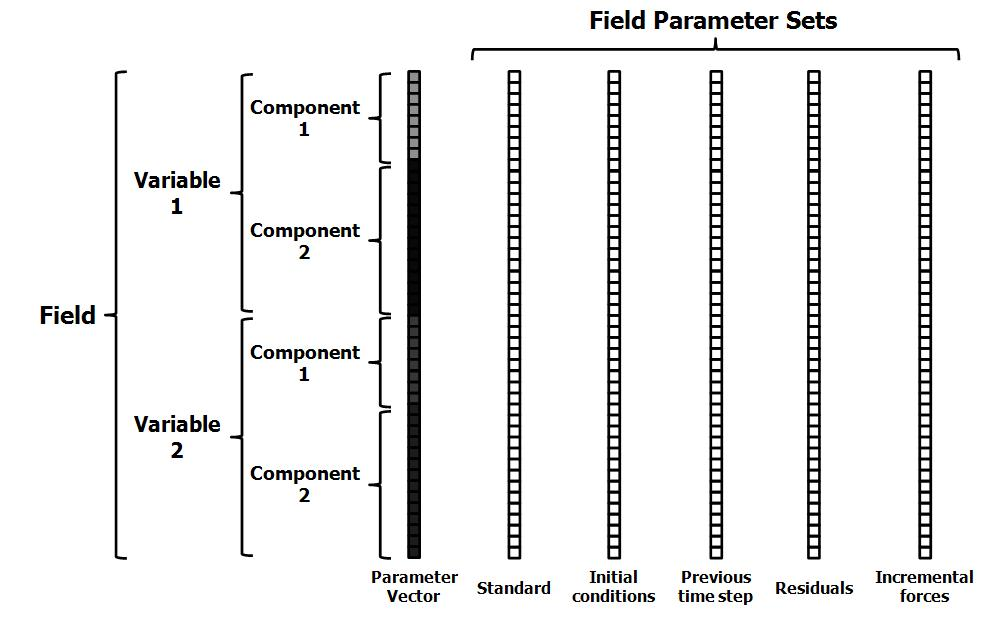Parameter set has the following Attributes:

• Set index
• Set type
• Parameters pointer

# Equation Sets

Equations sets are aimed to have multiple classes, e.g. Elasticity, Fluid mechanics, Electromagnetics, General field problems, Fitting, Optimisation. Different equations are within each class, e.g. Bidomain, Navier-stokes etc. Each equation can use different solution techniques, e.g. FEM, BEM, FD, GFEM. The equation set is associated with a region and is built using the fields defined on the region.

The numerical methods are used which will result in a discretised matrix-vector form of the governing equations. openCMISS is designed to generate equations sets with a number of "equations" matrices.

e.g, damped mass spring system Mu + Cu + Ku = f will be represented as: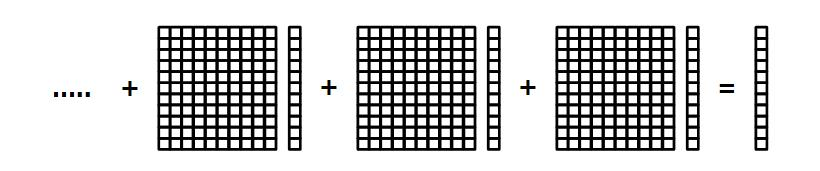Equations Set has the following attributes:

• User number
• Global number
• Finished tag
• Region pointer
• Class identifier
• Type identifier
• Sub type identifier
• Linearity type(?)
• Time dependence type(?)
• Solution method
• Geometry (fibre?) field pointer
• Materials field pointer
• Source field pointer
• Dependent field pointer
• Analytic info pointer(Analytic info stored in dependent field currently)
• Fixed conditions
• Equations pointer## Equations

Equation holds the matrices and mapping information.

The Field variable to matrix mappings maps each field variable onto the equations matrices or RHS vector.

e.g. Laplace(FEM): 2 variables, 1 component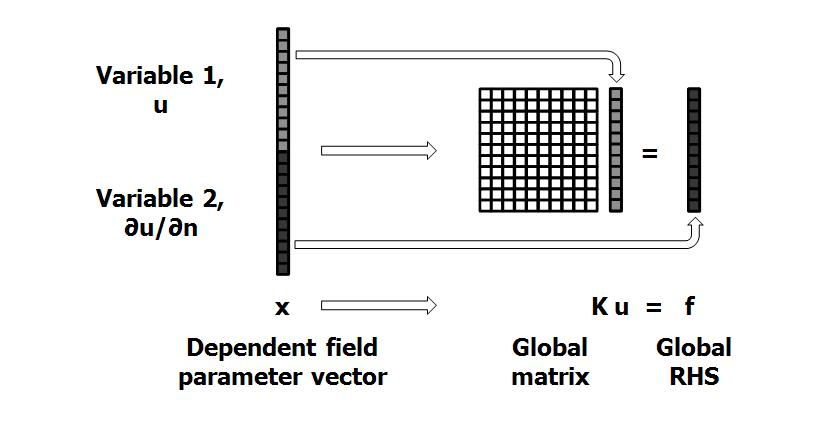e.g. Laplace(BEM): 2 variables, 1 component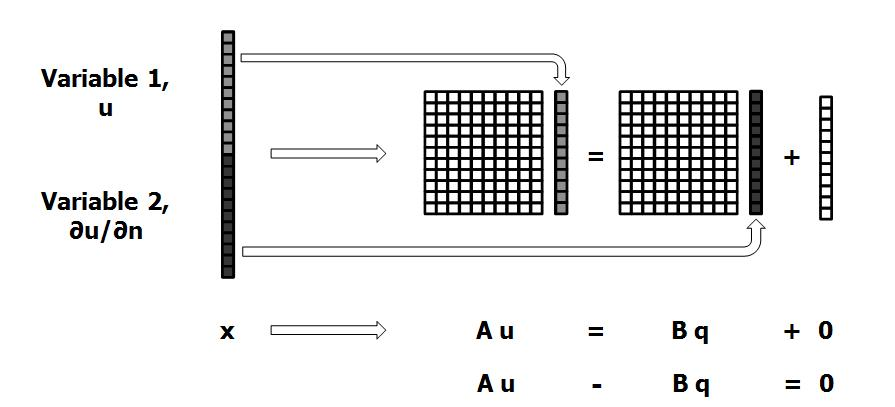e.g. Heat equation(explicit time/FEM space): 2 variables, 1 componentTODO matrix distribution

Equations has the following attributes:

• Equation set pointer
• Finished tag
• Output type
• Sparsity type
• Interpolation pointer
• Linear equation data pointer
• Nonlinear equation data pointer
• Time(non-static) data pointer
• Equations mapping pointer
• Equations Matrices